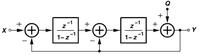# 2nd order Delta Sigma Modulator

Status
Not open for further replies.

#### Debdut

##### Full Member level 3I am creating a second order delta sigma modulator for a fractional n pll.
First I created the first order one. It worked ok when I removed the output flip flop that had to act as a comparator. Of course there's some sort of comparator glue logic. However it is combinational circuit. I wrote a code in matlab and it worked fine, designed the circuit in cadence and it worked fine. The comparator is showing repetitive output bit patterns that have an average value equal to the input, which is expected.

Now the main problem comes. When it comes to second order DSM, it is supposed to break the periodicity of the comparator output while still giving an average equal to the input.
I wrote the code for the following circuit in matlab, also I did some hand calculations for the following circuit. It is giving the correct average value. However there's still periodicity!!!!!!